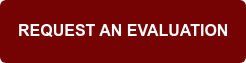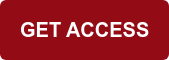# What is FEA (Finite Element Analysis) in CAD?

Fri Nov 22, 2019

Engineers have the unenviable position of being held entirely responsible for the integrity and safety of all the products and structures they design. Small mistakes in design often lead to imminent disasters (that frequently go to court and get litigated).

This is why products and structures go through an array of stress testing and optimization before being deployed in the real world. But doing so over hundreds of iterations (and to scale) can be prohibitively expensive. So engineers often look to simulation modeling techniques (like Finite Element Analysis) to automate and simplify this testing.

This helps to reduce the use of materials for iterative prototyping, which would otherwise be used in actualization.

Finite element analysis is one of the most common techniques used by engineers and CAD designers to simulate “stress” on their designs.

## What is FEA (Finite Element Analysis)?

Finite element analysis (FEA) is a numerical technique used to solve engineering problems with an array of physics-based calculations.

For example, an engineer designing a bridge will need to predict the behavior of the structure under load. The system of equations describing the distribution of structural stresses may be known, but cannot be easily (or directly) solved for a complicated structure such as a bridge.

Therefore, the system has to be broken down into small elements (the “finite elements”), whose calculations can be easily made and solved.

Finite elements can be one, two or three dimensional.

## Principles of Finite Element Analysis and Simulation Software

Finite Element method is generally used in FEA to compute approximate solutions of boundary problems (field problems) in engineering.

“The field” normally represents a physical structure as the main domain.

“Field variables” are the variables of interest that are governed by a differential equation. They must achieve a boundary condition for specific values of the variables (or related variables)

A “node” is a specific point on the finite element that contains the value of the field variable -- that is normally explicitly calculated. These values are then used to compute values at non-nodal points through interpolation of values at the nodes using shape functions.

## A general process in FEA

### 1. Preprocessing for Manufacturing

This stage requires you to define the domain problem, material properties, geometric properties, mesh model, boundary conditions and the element types. Typically at this stage, the engineer will simplify the problem as much as possible to get quick yet accurate results.

For example, prior to simulation, a CAD model may have lots of fillets, holes, or other features that aren’t essential to the simulation -- but features that hog computational resources and increase render times. Engineers typically remove these features to speed up their simulations.

This can be done manually (with designers going in and removing these features one by one) -- or automatically using CAD defeaturing on Spatial software. One big advantage of automating this process is the reduction of human error.### Spatial's 3D InterOp and Modeler Solutions Enable Feature Recognition and easy Defeaturing### 2. Solution

Here, computations are made for the unknown values of the field variables. You could use these values to compute derived variables such as stresses and reaction forces.

### 3. Post-processing

Sorting, plotting, and printing is the final set of activities where your selected results are implemented as solutions to your finite element analysis.

## Types of finite element method

Shortcomings in FEA modeling for some fields (such as fluid mechanics) have called for several improvements to the solution process while enhancing the diversity in the application of finite element analysis.

Some of the common types include;

• The extended finite method (XFEM)

Problems such as damage fracture or failure cannot be handled by the finite element method directly.

XFEM — which uses the Galerkin method and Heaviside step functions -- enables expansion of shape functions. The nodes are each given extra degrees of freedom at the points of discontinuity for consideration.

• The generalized finite element method

In this type, shape functions are multiplied by the partition-of-unity while being defined by global coordinates. This prevents re-meshing while you can form local element functions.

## Finite Element Analysis Applications in CAD

FEA is among the most powerful and commonly used features in CAD design.

It is integral to CAD for analyzing an object through the use of finite elements that include small rectangular and triangular shapes. To analyze the whole object, each finite element in the whole object network is analyzed, and the outcomes are combined to plot the overall behavior of a complex object. Each element can be analyzed for certain property thresholds like stress-strain, dynamics, thermal properties — or any other characteristics (depending on where it is applied).

In a CAD workflow, you can define each element and network structure as you require in your design.

## Why FEA software is useful

FEA allows engineers to conduct the simulation of a designed model rather than having to create a physical model for testing purposes.

FEM during analysis allows modeling of a diverse number of material types, and the ability to monitor how confined effects impact a small area of design in complicated geometry.

Engineers can use software specialized for finite element modeling for a wide range of tasks.

Fluid dynamics, deformation of a vehicle from collision and stress on human bones are some of the practical utilization of finite element modeling software by engineers.

## The Bottom line

FEA is predictive but not affirmative.

It does not ascertain that a design will withstand the simulated stresses with certainty. It only gives engineers a view of how the design may react to the imposed stresses while cutting on the cost of physical testing.

For the overall FEA experience, there are a number of CAD software with this feature that could help you design better and accurate structures in whichever field of engineering you are in.## You May Also Like

These Stories on CGM Modeler